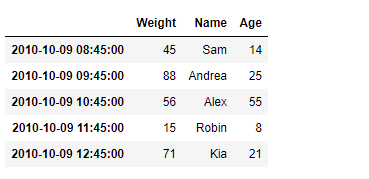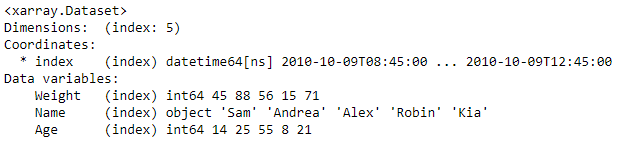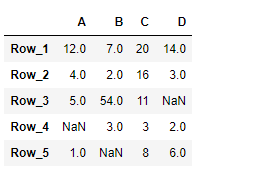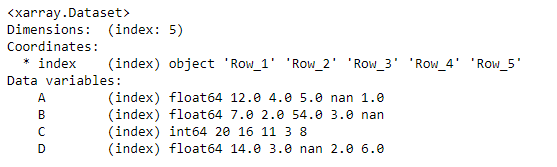Related Articles
Python | Pandas DataFrame.to_xarray
• Last Updated : 21 Feb, 2019

Pandas DataFrame is a two-dimensional size-mutable, potentially heterogeneous tabular data structure with labeled axes (rows and columns). Arithmetic operations align on both row and column labels. It can be thought of as a dict-like container for Series objects. This is the primary data structure of the Pandas.

Pandas` DataFrame.to_xarray()` function return an xarray object from the pandas object.

Syntax: DataFrame.to_xarray()

Parameter : None

Returns : xarray.DataArray or xarray.Dataset

Example #1: Use `DataFrame.to_xarray()` function to construct an xarray object using the given dataframe.

 `# importing pandas as pd``import` `pandas as pd`` ` `# Creating the DataFrame``df ``=` `pd.DataFrame({``'Weight'``:[``45``, ``88``, ``56``, ``15``, ``71``],``                   ``'Name'``:[``'Sam'``, ``'Andrea'``, ``'Alex'``, ``'Robin'``, ``'Kia'``],``                   ``'Age'``:[``14``, ``25``, ``55``, ``8``, ``21``]})`` ` `# Create the index``index_ ``=` `pd.date_range(``'2010-10-09 08:45'``, periods ``=` `5``, freq ``=``'H'``)`` ` `# Set the index``df.index ``=` `index_`` ` `# Print the DataFrame``print``(df)`

Output :Now we will use `DataFrame.to_xarray()` function to construct an xarray object using the given Dataframe.

 `# return an xarray object``result ``=` `df.to_xarray()`` ` `# Print the result``print``(result)`

Output :As we can see in the output, the `DataFrame.to_xarray()` function has successfully constructed an xarray object using the given dataframe.

Example #2: Use `DataFrame.to_xarray()` function to construct an xarray object using the given dataframe.

 `# importing pandas as pd``import` `pandas as pd`` ` `# Creating the DataFrame``df ``=` `pd.DataFrame({``"A"``:[``12``, ``4``, ``5``, ``None``, ``1``], ``                   ``"B"``:[``7``, ``2``, ``54``, ``3``, ``None``], ``                   ``"C"``:[``20``, ``16``, ``11``, ``3``, ``8``], ``                   ``"D"``:[``14``, ``3``, ``None``, ``2``, ``6``]}) `` ` `# Create the index``index_ ``=` `[``'Row_1'``, ``'Row_2'``, ``'Row_3'``, ``'Row_4'``, ``'Row_5'``]`` ` `# Set the index``df.index ``=` `index_`` ` `# Print the DataFrame``print``(df)`

Output :Now we will use `DataFrame.to_xarray()` function to construct an xarray object using the given Dataframe.

 `# return an xarray object``result ``=` `df.to_xarray()`` ` `# Print the result``print``(result)`

Output :As we can see in the output, the `DataFrame.to_xarray()` function has successfully constructed an xarray object using the given dataframe.

Attention geek! Strengthen your foundations with the Python Programming Foundation Course and learn the basics.

To begin with, your interview preparations Enhance your Data Structures concepts with the Python DS Course.

My Personal Notes arrow_drop_up